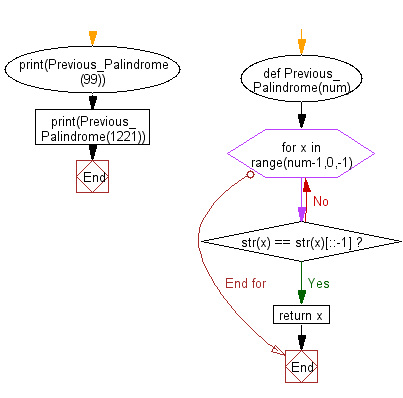﻿ Python Math: Find the next previous palindrome of a specified number - w3resource# Python Math: Find the next previous palindrome of a specified number

## Python Math: Exercise-23 with Solution

Write a python program to find the next previous palindrome of a specified number.

A palindromic number or numeral palindrome is a number that remains the same when its digits are reversed. Like 15951, for example, it is "symmetrical". The term palindromic is derived from palindrome, which refers to a word (such as " REDIVIDER" or even "LIVE EVIL") whose spelling is unchanged when its letters are reversed.

Sample Solution:-

Python Code:

``````def Previous_Palindrome(num):
for x in range(num-1,0,-1):
if str(x) == str(x)[::-1]:
return x
print(Previous_Palindrome(99));
print(Previous_Palindrome(1221));
```
```

Sample Output:

```88
1111
```

Pictorial Presentation:Flowchart:## Visualize Python code execution:

The following tool visualize what the computer is doing step-by-step as it executes the said program:

Python Code Editor:

Have another way to solve this solution? Contribute your code (and comments) through Disqus.

What is the difficulty level of this exercise?

Test your Programming skills with w3resource's quiz.

﻿

```>>> students = [{'name': 'John', 'score': 98}, {'name': 'Mike', 'score': 94}, {'name': 'Jennifer', 'score': 99}]